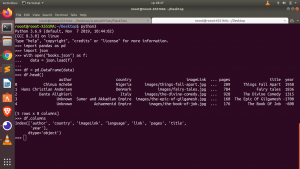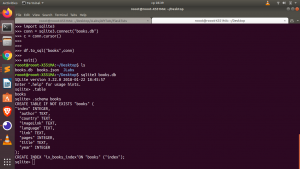# How to Convert JSON to SQL format In Python

In this tutorial we will see how to convert JSON – Javascript Object Notation to SQL data format such as sqlite   or db.

We will be using Pandas for this.

Installation

`pip install pandas sqlalchemy`
##### Method 1 : Using Sqlite3
```import pandas as pd
import json
import sqlite
# Open JSON data
with open("datasets.json") as f:

# Create A DataFrame From the JSON Data
df = pd.DataFrame(data)```Now we need to create a connection to our sql database. We will be using sqlite for that.

`import sqlite3conn = sqlite3.connect("data.db")c = conn.cursor()`

We can now convert our JSON data from dataframe to sqlite format such as db or sqlite.

`df.to_sql("tablename",conn)`

Note: The first argument is the table name you will be storing your data in.

##### Method 2 : Using SQLalchemy

We can also use the sqlalchemy  instead of sqlite3.

```from sqlalchemy import create_engine
engine = create_engine("sqlite:///my_data.db")
df.to_sql("table_name",conn=engine)```

In case you want to automatically get the columns and their datatype in sqlite for creating a class model schema, you can use the .schema option```sqlite3 my_data.db
>>> .table
>>> .schema book```

You can also check the video tutorial here